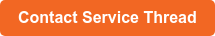## ISO 9001:2015 Standard RegisteredBonded thread size is communicated in various ways, mainly by ticket number and tex size. Although it’s easy to get caught up in the lingo and confuse the two, the ticket number and tex size are distinct characteristics of a bonded thread.

Here’s a look at what each of these terms means, as well as some tips on understanding the differences between them.

## Tex Size

The term “tex size” most likely derives from the word “textile” and is a universal measurement that allows consistency in sizing. The International Organization for Standardization (IOS), a global consortium of standards experts, prefers tex sizing for yarn and thread over other methods of measurement.

The tex size of thread is just one of several numbering systems that are classified as either direct or indirect systems. Tex falls under the direct system. It is the weight of a fixed-length rather than the length of a fixed weight (indirect).

The higher the tex number, the heavier the thread.

Tex size is the weight in grams of 1,000 meters of thread. For example, if 1,000 meters of a thread weighs 1 gram, tex size = 1.

So if 1,000 meters of a particular thread weighs 30 grams, it would be labeled tex 30, and if it weighs 35 grams, it would be tex 35, and so forth.

Keep in mind that 1,000 meters of one individual weight-type (lightweight, heavy-duty, etc.) of yarn will not weigh the same as 1,000 meters of another type, so they will have different tex sizes even though they are the same length and fiber. So a medium-weight cotton at 1,000 meters might be tex 25, while a heavy-duty cotton at 1,000 meters might be tex 72.

## Ticket Size

Ticket size is a manufacturer’s reference to the final denier size of a given thread, with denier being the weight in grams of 9,000 meters of thread. However, it’s not about the single’s denier size alone.

When you figure ticket size, you also have the impact of multiple thread plies, level of singles, ply twist, and overall twist contraction, which reduces the length and adds to the denier. So, to give a reference point for denier and ticket size, the rule of thumb is:

Singles denier x number of plies x 0.11 = ticket size.  For example 1680 x 3 x 0.11 = ticket size #554.

Ticket size is not an exact calculation and due to minor differences in denier between substrates, different final denier sizes may lend to the same final ticket size.  Let’s look at an example of this.

A polyester that is ticket size 69 is made of 3 plies of 220 denier each, total 660 denier x 0.11 = 72.6. A nylon that is ticket size 69 is made up of 3 plies of 210 denier, which equals 630 denier x 0.11 = 69.3. The same ticket size will be given to both and they will also be given the same tex size of 70.

## Ticket Number

A ticket number is yet another reference to the size of a thread. Ticket numbers typically resemble the fixed weight system, whereby the higher the number the finer the thread, and lower numbers will be thicker threads.

To arrive at a ticket number from a tex size, use the following calculation: 1000/tex size x 3

For example 1000/Tex 40 x 3 = ticket 75.

## Ordering by Ticket Number, Ticket Size, and Tex Size

Although ticket numbers, ticket sizes, and tex sizes can be a bit tricky until you get used to them, it soon becomes second nature to look at these numbers for information about the industrial thread you purchase. You can always use Service Thread’s conversion calculator to quickly switch between common thread types and sizes.

If you need more information, we’re here to help. It’s vital to make the right choice for your specific application and industrial processing setup. For more assistance with making the right thread selections, contact Service Thread.